Function Repository Resource:

# MixtilinearIncircles

Get the three mixtilinear incircles of a triangle

Contributed by: Shenghui Yang
 ResourceFunction["MixtilinearIncircles"][{p1,p2,p3}] gives the three mixtilinear incircles for a triangle with vertices p1,p2 and p3. ResourceFunction["MixtilinearIncircles"][{p1,p2,p3},property] returns additional related geometric objects of the mixtilinear incircles for the given property. ResourceFunction["MixtilinearIncircles"][{p1,p2,p3},{prop1,…}] returns a list of properties.

## Details

The mixtilinear incircles of a triangle are the three circles that are tangent to two sides and internally tangent to the circumcircle.
Given triangle ABC, the convention of the order for mixtilinear incenters and circles follows the diagram given here (points and circles are sorted with respect to alphabetic order in subscript):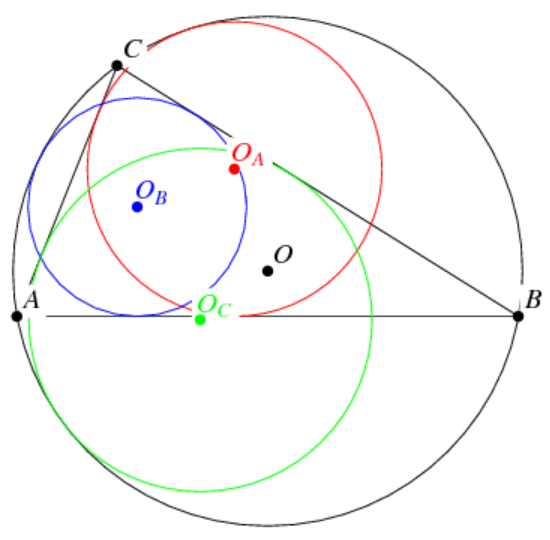Given triangle ABC, the mixtilinear radical axis for vertex A is the line through the intersection of circles OB and OC in the diagram. Those for vertices B and C are defined similarly.
Given triangle ABC, the mixtilinear circle passes through points OA, OB and OC.
The following values are available for property:
 "Circles" the mixtilinear incircles of a triangle "MixtilinearIncircles" same as "Circles" "MixtilinearIncenters" the centers of mixtilinear incircles of a triangle "MixtilinearInradii" the radii of mixtilinear incircles of a triangle "MixtilinearTriangle" the triangle connecting the three mixtilinear incenters "MixtilinearCircle" the circumcircle of the mixtilinear triangle "MixtilinearRadicalAxes" the radical axes of pairs of the mixtilinear incircles "MixtilinearRadicalCenter" the intersection of the radical axes "ContactsOnCirumcircle" the contacts between mixtilinear incircles and circumcircle "OverlappingInversions" combination of circle inversion and reflection along the angle bisector "X56" the concurrence of lines joining vertices and contacts on the circumcircle All an association including all properties given above
ResourceFunction["MixtilinearIncircles"][Triangle[{p1,p2,p3}]] is equivalent to ResourceFunction["MixtilinearIncircles"][{p1,p2,p3}].

## Examples

### Basic Examples (2)

Find the three mixtilinear incircles for a reference triangle:

 In:=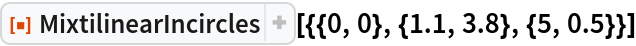Out=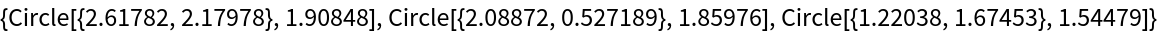An equivalent specification:

 In:=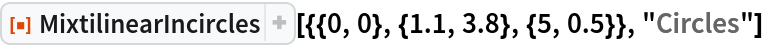Out=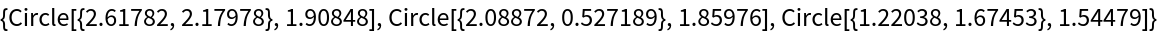### Scope (4)

Find a list of properties for a triangle:

 In:=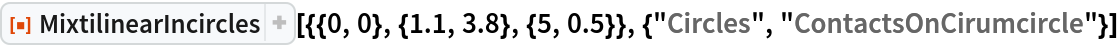Out=Visualize the three mixtilinear circles along with the reference triangle:

 In:=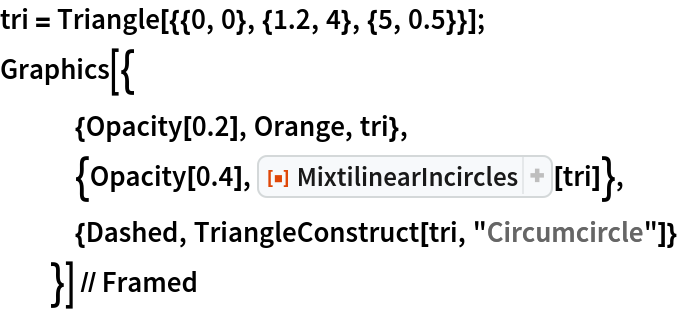Out=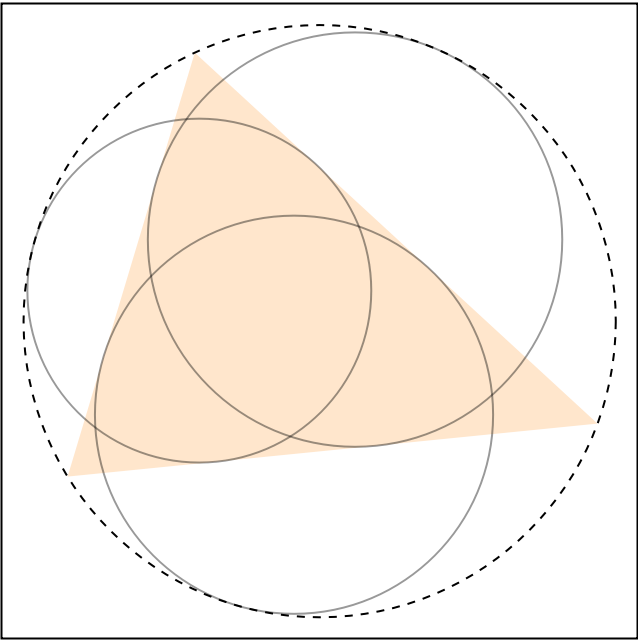MixtilinearIncircles works with Triangle objects:

 In:=Out=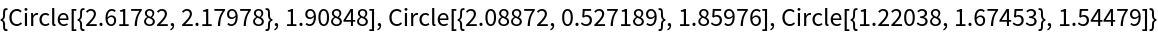Find the mixtilinear incircles for several triangles:

 In:=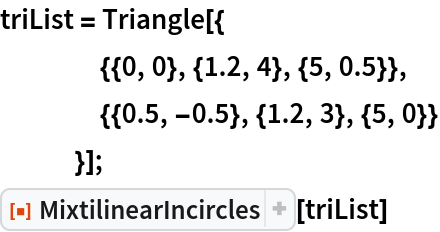Out=### Applications (3)

In the leftmost diagram, the overlapping inversion for vertex A={0,0} uses circle inversion at A with power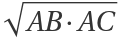and then applies the reflection transformation about the angular bisector of A. This operation sends the mixtilinear incircle and circumcircle contact point (black) to the blue point, which is the contact point between A-excircle and side BC:

 In:=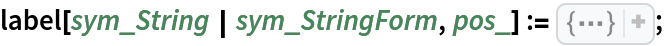In:=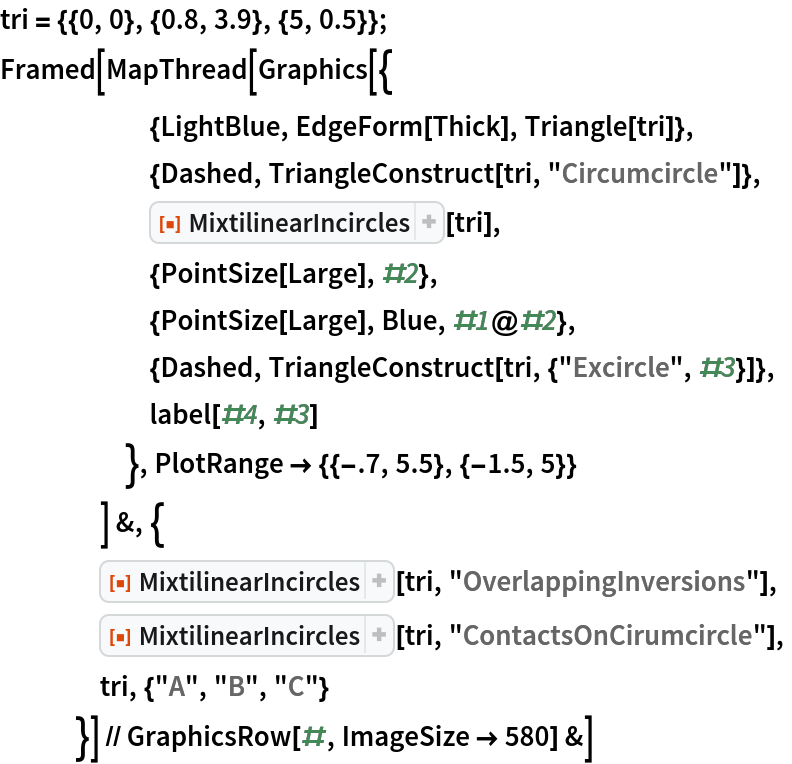Out=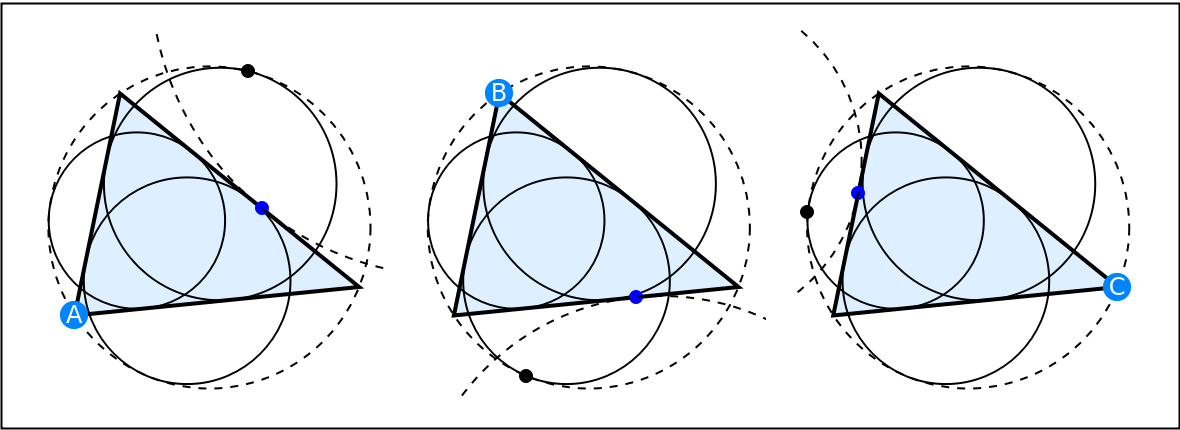Therefore, the lines connecting A to the blue point and A to the black point are isogonal conjugate with respect to the angle bisector of A. Similar results apply to B and C.

The isogonal conjugacy also relates the Nagel point (brown) and the Kimberling center X56 (blue). The latter is the concurrence of the lines joining the vertex and the corresponding mixtilinear incircle-to-circumcircle contact point:

 In:=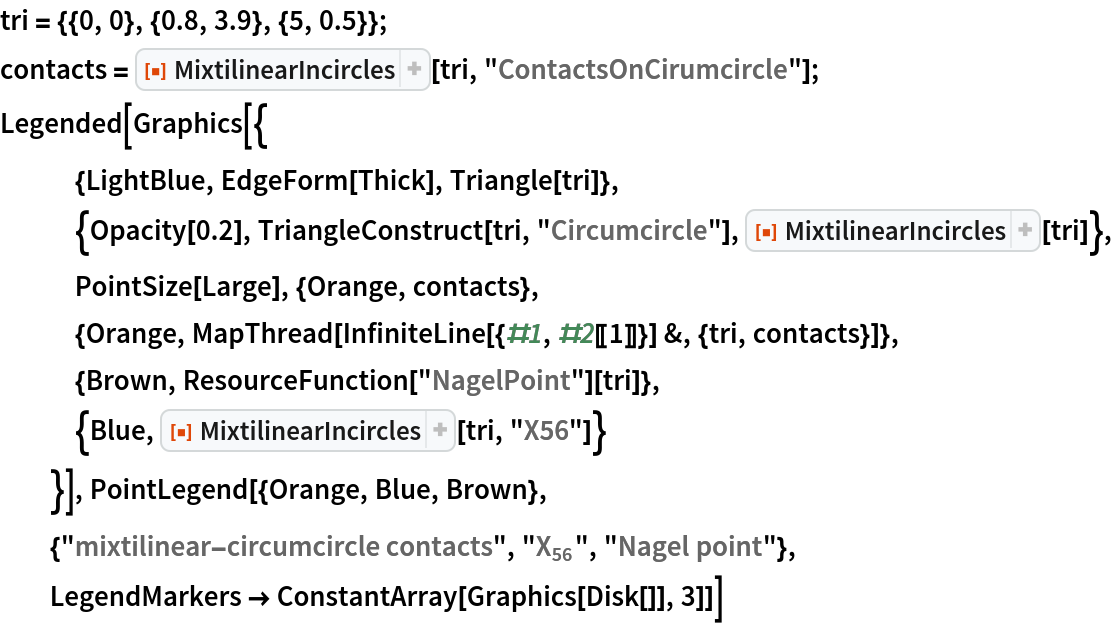Out=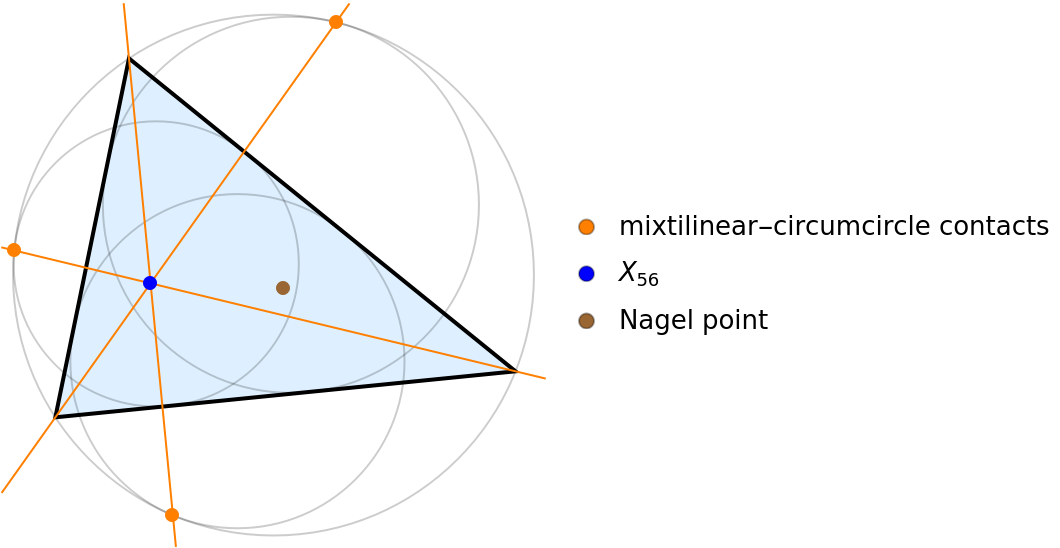Given triangle ABC, the overlapping inversion at A sends the incenter to the A-excenter. Similar results apply to B and C:

 In:=Out=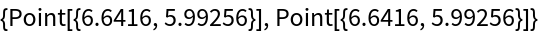### Properties and Relations (1)

Display all available information from MixtilinearIncircles in a dataset:

 In:=Out=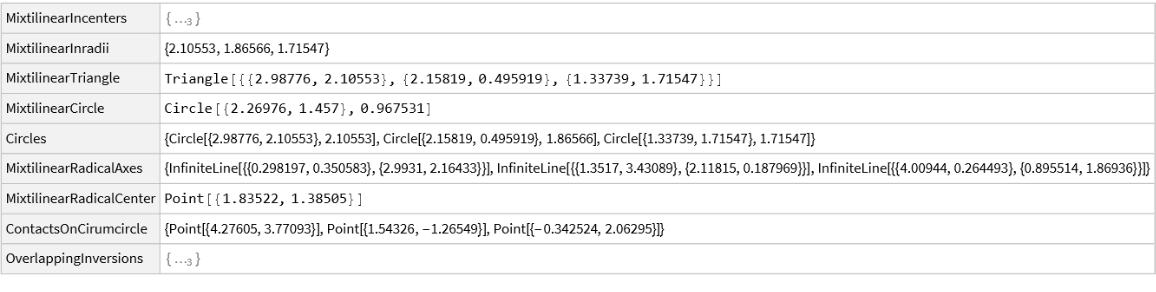### Neat Examples (3)

For a given triangle, the point X56 (blue), mixtilinear radical center (red), incenter (orange) and circumcenter (black) are collinear:

 In:=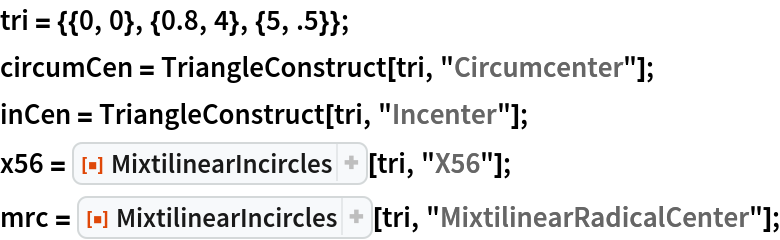Show the collinearity:

 In:=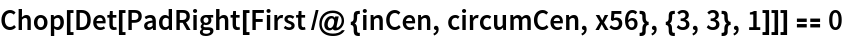Out=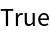In:=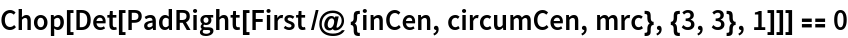Out=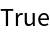Visualize the points and the line passing the four points:

 In:=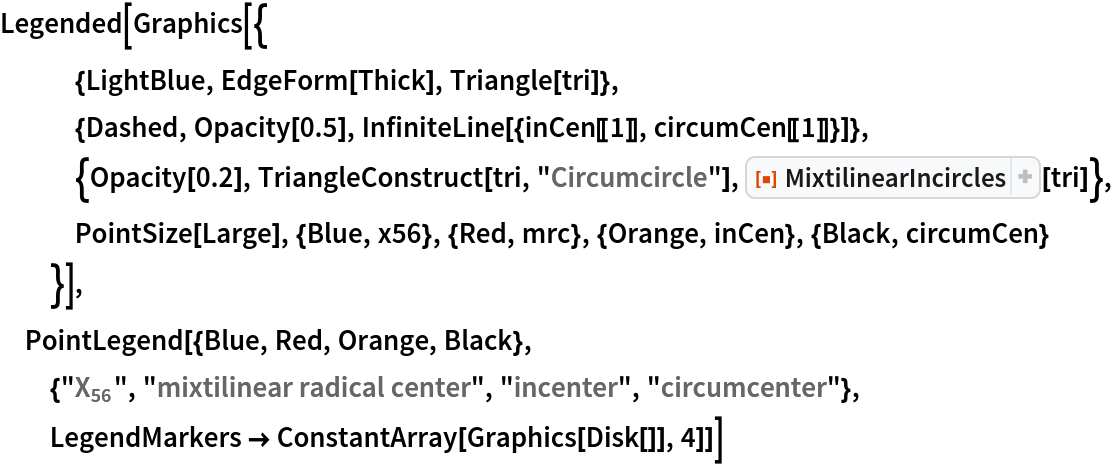Out=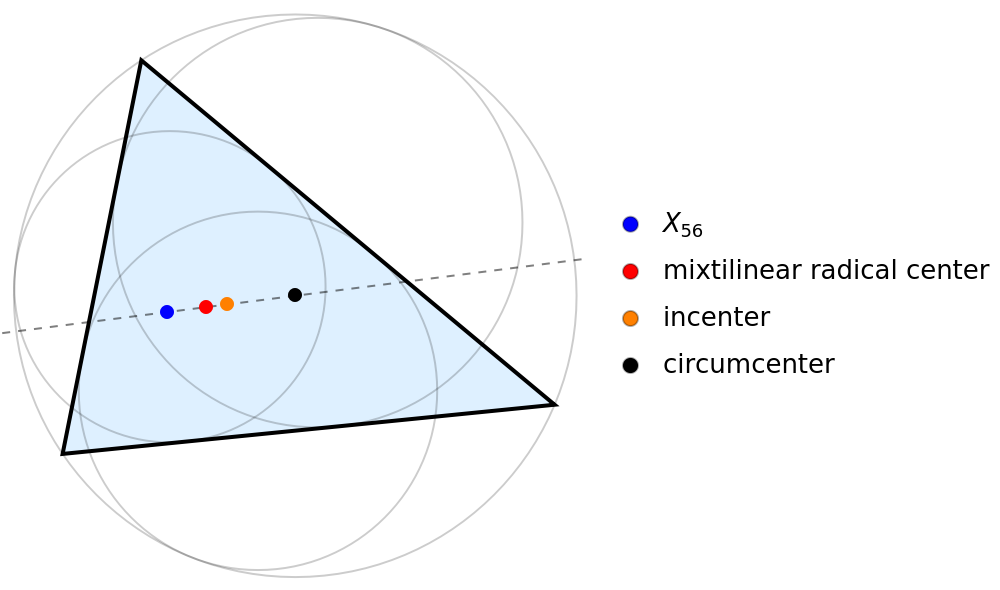Shenghui Yang

## Version History

• 1.0.1 – 22 April 2022
• 1.0.0 – 01 November 2021